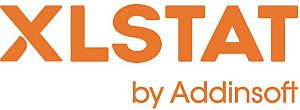# XLSTAT - Correlation Tests

## Correlation tests in XLSTAT

XLSTAT proposes three correlation coefficients to compute the correlation between a set of quantitative variables, whether continuous, discrete or ordinal:

### Pearson correlation coefficient

This coefficient corresponds to the classical linear correlation coefficient. This coefficient is well suited for continuous data. Its value ranges from -1 to 1, and it measures the degree of linear correlation between two variables.

Note: the squared Pearson correlation coefficient gives an idea of how much of the variability of a variable is explained by the other variable. The p-values that are computed for each coefficient allow testing the null hypothesis that the coefficients are not significantly different from 0. However, one needs to be cautious when interpreting these results, as if two variables are independent, their correlation coefficient is zero, but the reciprocal is not true.

### Spearman correlation coefficient (rho)

This coefficient is based on the ranks of the observations and not on their value. This coefficient is adapted to ordinal data. One can interpret this coefficient in terms of explained variability of the ranks.

### Kendall correlation coefficient (tau)

It is well suited for ordinal variables as it is based on ranks. It can be interpreted in terms of probability - it is the difference between the probabilities that the variables vary in the same direction and the probabilities that the variables vary in the opposite direction. When the number of observations is lower than 50 and when there are no ties, XLSTAT gives the exact p-value. If not, an approximation is used. The latter is known as being reliable when there are more than eight observations.This analysis is available in the XLStat-Basic addin for Microsoft ExcelKovach Computing Services (KCS) was founded in 1993 by Dr. Warren Kovach. The company specializes in the development and marketing of inexpensive and easy-to-use statistical software for scientists, as well as in data analysis consulting.

### Get in Touch

• Email:
sales@kovcomp.com